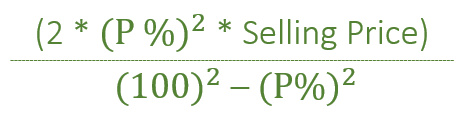# Loss when two items are sold at same price and same percentage profit/loss

Given the Selling price i.e ‘SP’ of the two items each. One item is sold at ‘P%’ Profit and other at ‘P%’ Loss. The task is to find out the overall Loss.

Examples:

```Input: SP = 2400, P = 30%
Output: Loss = 474.725

Input: SP = 5000, P = 10%
Output: Loss = 101.01
```

## Recommended: Please try your approach on {IDE} first, before moving on to the solution.

Approach:How does the above formula work?

For profit making item :
With selling price (100 + P), we get P profit.
With selling price SP, we get SP * (P/(100 + P)) profit

For loss making item :
With selling price (100 – P), we get P loss.
With selling price SP, we get SP * (P/(100 – P)) loss

Net Loss = Total Loss – Total Profit
= SP * (P/(100 – P)) – SP * (P/(100 + P))
= (SP * P * P * 2) / (100*100 – P*P)

Note: The above formula is applicable only when the Cost price of both the items are different. If CP of both the items are same then, in that case, there is ‘No profit No loss’.

Below is the implementation of the above approach

## C++

 `// C++ implementation of above approach. ` `#include ` `using` `namespace` `std; ` ` `  `// Function that will ` `// find loss ` `void` `Loss(``int` `SP, ``int` `P) ` `{ ` ` `  `    ``float` `loss = 0; ` ` `  `    ``loss = (2 * P * P * SP) / ``float``(100 * 100 - P * P); ` ` `  `    ``cout << ``"Loss = "` `<< loss; ` `} ` ` `  `// Driver Code ` `int` `main() ` `{ ` `    ``int` `SP = 2400, P = 30; ` ` `  `    ``// Calling Function ` `    ``Loss(SP, P); ` ` `  `    ``return` `0; ` `} `

## Java

 `// Java implementation of above approach. ` `class` `GFG  ` `{ ` ` `  `// Function that will ` `// find loss ` `static` `void` `Loss(``int` `SP, ``int` `P) ` `{ ` ` `  `    ``float` `loss = ``0``; ` ` `  `    ``loss = (``float``)(``2` `* P * P * SP) / (``100` `* ``100` `- P * P); ` ` `  `    ``System.out.println(``"Loss = "` `+ loss); ` `} ` ` `  `// Driver Code ` `public` `static` `void` `main(String[] args)  ` `{ ` `    ``int` `SP = ``2400``, P = ``30``; ` ` `  `    ``// Calling Function ` `    ``Loss(SP, P); ` `} ` `} ` ` `  `// This code has been contributed by 29AjayKumar `

## Python3

 `# Python3 implementation of above approach.  ` ` `  `# Function that will find loss  ` `def` `Loss(SP, P):  ` `     `  `    ``loss ``=` `0` `    ``loss ``=` `((``2` `*` `P ``*` `P ``*` `SP) ``/`  `            ``(``100` `*` `100` `-` `P ``*` `P))  ` `    ``print``(``"Loss ="``, ``round``(loss, ``3``))  ` ` `  `# Driver Code  ` `if` `__name__ ``=``=` `"__main__"``: ` ` `  `    ``SP, P ``=` `2400``, ``30` ` `  `    ``# Calling Function  ` `    ``Loss(SP, P)  ` ` `  `# This code is contributed by Rituraj Jain `

## C#

 `// C# implementation of above approach. ` `class` `GFG  ` `{ ` ` `  `// Function that will ` `// find loss ` `static` `void` `Loss(``int` `SP, ``int` `P) ` `{ ` ` `  `    ``double` `loss = 0; ` ` `  `    ``loss = (``double``)(2 * P * P * SP) / (100 * 100 - P * P); ` ` `  `    ``System.Console.WriteLine(``"Loss = "` `+  ` `                            ``System.Math.Round(loss,3)); ` `} ` ` `  `// Driver Code ` `static` `void` `Main()  ` `{ ` `    ``int` `SP = 2400, P = 30; ` ` `  `    ``// Calling Function ` `    ``Loss(SP, P); ` `} ` `} ` ` `  `// This code has been contributed by mits `

## PHP

 ` `

Output:

```Loss = 474.725
```

Attention reader! Don’t stop learning now. Get hold of all the important DSA concepts with the DSA Self Paced Course at a student-friendly price and become industry ready.

My Personal Notes arrow_drop_upCheck out this Author's contributed articles.

If you like GeeksforGeeks and would like to contribute, you can also write an article using contribute.geeksforgeeks.org or mail your article to contribute@geeksforgeeks.org. See your article appearing on the GeeksforGeeks main page and help other Geeks.

Please Improve this article if you find anything incorrect by clicking on the "Improve Article" button below.